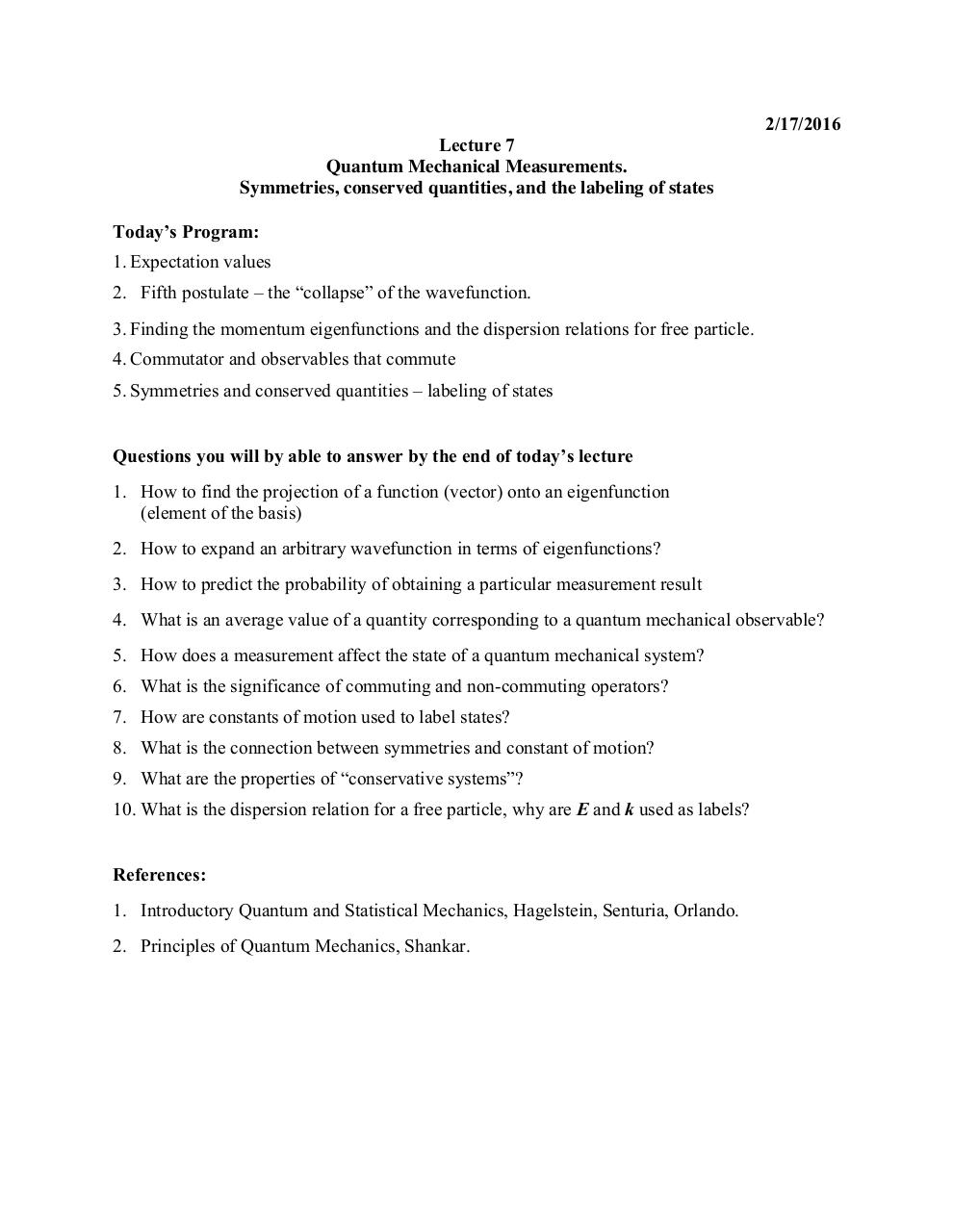# L7+QM+Measurements+and+Constants+of+Motion.pdfPage 1 2 3 4 5 6 7 8 9

#### Text preview

2/17/2016
Lecture 7
Quantum Mechanical Measurements.
Symmetries, conserved quantities, and the labeling of states
Today’s Program:
1. Expectation values
2. Fifth postulate – the “collapse” of the wavefunction.
3. Finding the momentum eigenfunctions and the dispersion relations for free particle.
4. Commutator and observables that commute
5. Symmetries and conserved quantities – labeling of states
Questions you will by able to answer by the end of today’s lecture
1. How to find the projection of a function (vector) onto an eigenfunction
(element of the basis)
2. How to expand an arbitrary wavefunction in terms of eigenfunctions?
3. How to predict the probability of obtaining a particular measurement result
4. What is an average value of a quantity corresponding to a quantum mechanical observable?
5. How does a measurement affect the state of a quantum mechanical system?
6. What is the significance of commuting and non-commuting operators?
7. How are constants of motion used to label states?
8. What is the connection between symmetries and constant of motion?
9. What are the properties of “conservative systems”?
10. What is the dispersion relation for a free particle, why are E and k used as labels?
References:
1. Introductory Quantum and Statistical Mechanics, Hagelstein, Senturia, Orlando.
2. Principles of Quantum Mechanics, Shankar.